GDXXRW

GDXXRW is a utility to read and write Excel spreadsheet data. GDXXRW can read multiple ranges in a spreadsheet and write the data to a GDX file, or read from a GDX file, and write the data to different ranges in a spreadsheet.

Note
GDXXRW is available on Windows only. This is an important factor to consider when moving an existing code to GAMS Engine.

# Usage

gdxxrw inputFile {outputFile} {options} [symbols]


Options and symbol specifications can also be read from a text file; the use of an option file is indicated by preceding the file name with a @ (At sign.). When reading from a text file, lines starting with an asterisk (*) will be ignored and act as a comment.

Options and symbol specifications can also be read from an area in a spreadsheet; see index below.

Files without a full path name are assumed to be in the current directory when using a command prompt. When using the GAMS IDE, these files are assumed to be in the current project directory. The use of file names with embedded blanks is allowed as long as the file name is enclosed in double-quotes (").

Note
A small utility program is available to see if Excel is installed, to close an Excel file etc. See XLSTALK for more details. To read data from an Excel file without Excel installed see XLSDUMP.

# Options

Describing the actions to be taken by GDXXRW requires passing a number of options and symbol specifications to the program. The ability of GDXXRW to process multiple actions in a single call makes the option passing and interpretation more complex.

There are four kinds of options:

1. Immediate Immediate options are recognized and processed before any other actions are taken and can only be specified once. Examples are:

input= output= trace=

2. Global Global options are interpreted from left to right and affect every action that follows. The same option can be used multiple times to affect the actions that follow. Examples are:

skipEmpty= epsOut=

3. Symbol A symbol definition introduces a new action for reading or writing a symbol. Examples are:

par= set= dSet=

4. Symbol attributes Attributes specify additional information for the last symbol defined. Examples are:

dim= cDim= merge clear

## Immediate Options

Immediate options are recognized independent of their position on the command line. They are global and they can only be specified once.

Option Default Description
input none Specify the input filename (required).
output inputFileName Specify the output filename.
log none Specify the log filename.
logAppend none Appending the log information to the file specified.
index none Indicates reading the options and symbols directly from the spreadsheet.
password none Password for an encrypted input file.
rWait 0 Delay after opening a spreadsheet.
checkDate disabled Write GDX file only if the input file is more recent than the GDX file.
useRC disabled Use Row-Column notation to specify cells and ranges.
reCalc N Controls if recalculations of cells inside Excel are executed after writing to the spreadsheet.
trace 1 Controls the amount of information written to the log.
maxDupeErrors 0 Maximum number of duplicate records allowed for a symbol.
updLinks 0 Updating of cells that refer to other spreadsheets.
runMarcos 0 Execution of Excel Auto macros.

Some more detailed remarks on the immediate options:

input = fileName (required, default = none)

Synonym: i

Either use the keywords input or i to specify the input file name anywhere on the command line or just specify the input file name without keyword at the first position behind GDXXRW. The file extension of the input file is required and determines the action taken by the program.

The extension .gdx for the input file will read data from a GDX file and write data to a spreadsheet. The extension .xls, .xlsx, .xlsm, or .xlsb for the input file will read a spreadsheet and write the data to a .gdx file. In addition to the .xls, .xlsx, .xlsm, or .xlsb input file extension, the following file extensions are also valid for spreadsheet input files: .wk1, .wk2, .wk3 and .dbf.

A file sharing conflict will arise when writing to a spreadsheet with the target file open in Excel. Either close the file in Excel before executing GDXXRW, or mark the spreadsheet as a shared workbook in Excel. To change the shared status of a workbook, use the Excel commands available under: Tools|Share Workbook.

Writing to a shared workbook can be painfully slow; simply closing the file and reopen the file after GDXXRW has finished is often a better option.

output = fileName (default = inputFileName)

Synonym: o

When an output file is not specified, the output file will be derived from the input file by changing the file extension of the input file and removing any path information. The file type, i.e. the file extension, depends on the installed version of Excel. Versions prior to Excel 2007 use the .xls file extension, later version use .xlsx, .xlsm, and .xlsb. Excel 2007 can write .xls files, but in that case the output file has to be specified with an .xls file extension.

log = fileName (default = none)

Specifies the filename of the log file. When omitted, log information will be written to standard output. When using GDXXRW in a GAMS model that is started from the GAMS IDE, the output will be written to the IDE process window.

logAppend = fileName (default = none)

Using logAppend will add the log information to the end of the file specified. If the file does not exist yet, a new one will be created.

index = Excel Range

The index option is used to obtain the global options, symbols and symbol attributes specified by reading them from the spreadsheet directly. The parameters are read using the specified range, and treated as if they were specified directly on the command line. The first three columns of the range have a fixed interpretation: dataType, Symbol identifier and dataRange. The fourth and following columns can be used for additional parameters. The column header contains the keyword when necessary, and the cell content is used as the parameter value. See Reading Spreadsheet using the Index Option for instance.

password = string (default = none)

Specifies a password for a protected spreadsheet file.

rWait = integer (default = 0)

Introduce a delay (in milliseconds) after opening a spreadsheet before accessing the data. This parameter can be used to work around an issue we encountered that Excel indicated it was not ready. The issue can arise during the data exchange with Excel Sheets that contain macros, plots or pivot tables where GDXXRW attempts to access a sheet while Excel is busy updating macros, graphs, and pivot tables.

checkDate (disabled by default)

When specified, no data will be written if the output file already exists and the file date for the output file is more recent than the file date for the input file. Provides a simple check to update the output file only if the input file has changed to save resources.

useRC (disabled by default)

Specify that all cell and range references use RC notation. So, instead of specifying the range Sheet1!A1:D6, one specifies Sheet1!R1C1:R6C4. When tracing is enabled, ranges will be reported in RC notation. This is a global option and applies to all cell references.

reCalc = flag (default = N)

Enable or disable the recalculations of cells inside Excel after writing to the spreadsheet. If there are many formulas in the spreadsheet the recalculation of cells can become very expensive and slowing down the writing process. By default, the recalculation is disabled and can be enabled via this option.

trace = integer (default = 1)

Sets the amount of information written to the log (for a better debugging). Higher values will generate more output. Valid range is 0..4.

0   Minimal information is included in the output
1   Message appears telling about each GDXXRW call indicating input file, output file and execution time
2   Message appears giving the level 1 output plus a listing for each symbol specified, indicating the type, sheet name, dimension, data range and the range of the row and column headers
3   Message appears giving the level 2 output plus cell ranges affected by reading, writing and clearing
4   Message appears giving the level 3 output plus cell addresses, and numerical or string values for every cell worked with

maxDupeErrors = integer (default = 0)

Sets the maximum number of duplicate records that is allowed when reading a spreadsheet and writing to a GDX file. The duplicate records for each symbol will be reported in the logfile, and if their accumulated number does not exceed the maximum specified using this option, the GDX file will not be deleted. This is a global option and applies to each symbol read from the spreadsheet.

The option is demonstrated in Reading Set from Lists with Duplication.

updLinks = integer (default = 0)

Specifies how links in a spreadsheet should be updated. The valid range is 0..3.

0   Doesn't update any references
1   Updates external references but not remote references
2   Updates remote references but not external references
3   Updates both remote and external references

runMacros = integer (default = 0)

This option controls the execution of the 'Auto_open' and the 'Auto_close' macros when opening or closing a spreadsheet. Valid range is 0..3.

0   Doesn't execute any macros
1   Executes Auto_open macro
2   Executes Auto_close macro
3   Executes Auto_open and Auto_close macro

## Global Options

The following options affect the symbols that follow the option. They remain in effect unless they are redefined and used again for another symbol.

Option Default Description
acronyms 0 Controls the handling of acronyms.
cMerge 0 Controls the handling of merged Excel ranges.
dSetText N Read explanatory text for set elements of domain sets.
epsOut Eps String to be used when writing the value for 'Epsilon'.
filter 0 Set the Excel filter for symbols written to Excel.
incRC N Include Excel row and column indices when a symbol is written to the GDX file.
mInfOut -Inf String to be used when writing the value for 'Negative infinity'.
NaIn none String to be used when reading a value for 'Not available'.
nameConv N Controls the interpretation of an Excel range.
NaOut NA String to be used when writing the value for 'Not available'.
pInfOut +Inf String to be used when writing the value for 'Positive infinity'.
resetOut disabled Reset the output strings for special values to their defaults.
squeeze Y Controls writing of default values of sub-fields of variables and equations resp. the handling of zero values within parameters when reading from spreadsheet.
skipEmpty 1 Number of empty rows or columns indicating the end of a block when reading from spreadsheet using the top left cell specification.
UndfOut Undf String to be used when writing the value for 'Undefined'.
allUELs Y Controls the handling of UELs without associated values in the data range.
zeroOut 0 String to be used when writing the value for 'Zero'.

Some more detailed remarks on the global options:

acronyms = integer (default = 0)

A non-zero value indicates that acronyms can be expected and should be processed.

If no acronym processing takes place, reading an identifier in the data section of a sheet will generate an error. Writing an acronym to a sheet will write the internal numerical representation of the acronym.

Processing acronyms:

When reading a spreadsheet, an identifier in the data section of the sheet will be interpreted as an acronym and will be written to the GDX file.

When writing to a spreadsheet, a data tuple containing an acronym will be stored using the corresponding identifier of the acronym.

cMerge = integer (default = 0)

Option indicating how to read an empty cell that is part of a merged Excel range. See Reading merged Excel Ranges with cMerge. Possible values and their interpretation are:

0   Leave the cell empty
1   Use merged value in row and column headers only
2   Use merged value in all cells

dSetText = flag (default = N)

This controls the reading of explanatory text for set elements of domain sets. By default, no text is read for domain sets. If this option is activated this is changed. If an element appears more than once, the first one defines the explanatory text read.

epsOut = string (default = Eps)

String to be used when writing the value for 'Epsilon'. This option is demonstrated in Reading Special Values from Spreadsheet and writing to Spreadsheet and Writing Parameter to Spreadsheet including Zero Values.

filter = integer (default = 0)

Adds basic Excel filter to the columns of the spreadsheet to display only those values matching some conditions. Using this option when reading an Excel file will result in an error. Specifying filter=1 will set an Excel filter for the row of labels that are closest to the data values. When there are multiple rows in a column header (cDim > 1) we can specify filter=x where x is a number of the range 2..cDim, indicating to use a row farther away from the data values. See also Writing to Spreadsheet using a Filter.

incRC = flag (default = N)

Include Excel row and column indices when a symbol is written to the GDX file. For example, when we write a parameter P with indices I and J, without this option it will be written as P(I, J). When incRC is enabled, the parameter will be written as P(Excel_Rows, I, Excel_Columns, J). Note that the sets Excel_Rows and Excel_Columns will be added to the GDX file automatically.

mInfOut = string (default = -Inf)

String to be used when writing the value for 'Negative infinity'. This option is demonstrated in Reading Special Values from Spreadsheet and writing to Spreadsheet.

NaIn = string (default = none)

String to be used when reading a value for 'Not available'; this string is recognized in addition to the string 'NA' and is not case-sensitive. This option is demonstrated in Reading Special Values from Spreadsheet and writing to Spreadsheet.

nameConv = flag (default = N)

Synonym: nC

The naming convention parameter is used to change the interpretation of an Excel range that does not contain an '!' (exclamation mark). For details see Excel Ranges below.

NaOut = string (default = NA)

String to be used when writing the value for 'Not available'. This option is demonstrated in Reading Special Values from Spreadsheet and writing to Spreadsheet.

pInfOut = string (default = +Inf)

String to be used when writing the value for 'Positive infinity'. This option is demonstrated in Reading Special Values from Spreadsheet and writing to Spreadsheet.

resetOut (disabled by default)

Reset the output strings for special values to their defaults. This option is demonstrated in Reading Special Values from Spreadsheet and writing to Spreadsheet.

squeeze = flag (default = Y)

Synonym: sq

Writing to a spreadsheet:

The squeeze option affects the writing of sub-fields of variables and equations. A value for the field that is the default value for that type of variable or equation will not be written to the spreadsheet. For example, the default for .l (level value) is 0.0, and therefore zero will not be written to the spreadsheet. When we set squeeze=n, all values will be written to the spreadsheet.

The squeeze option for writing data is demonstrated in Reading Data from Spreadsheet and writing Data to Spreadsheet after Solve and Writing Parameter to Spreadsheet including Zero Values.

When the squeeze option is enabled, zero values for parameters will not be written to the GDX file. When the squeeze option is disabled, zero values will be written to the GDX file. In either case, empty cells, or cells containing blanks only, will never be written to the GDX file.

The squeeze option for reading data is demonstrated in Reading Special Values from Spreadsheet and writing to Spreadsheet.

skipEmpty = integer (default = 1)

Synonym: sE

The skipEmpty option can be used when reading a spreadsheet and the range is specified using the top left corner instead of a block range. The value defines the number of empty row or column cells signal the end of a block. Valid values are 0..n. If the range is specified using a block range, skipEmpty will be ignored. Blank rows or columns will be skipped automatically.

Note that skipEmpty is also valid when using the merge resp. clear options in order to write data to spreadsheet (in a specific order determined by matching row and column labels already stored in the spreadsheet).

UndfOut = string (default = Undf)

String to be used when writing the value for 'Undefined'. This option is demonstrated in Reading Special Values from Spreadsheet and writing to Spreadsheet.

allUELs = flag (default = Y)

When enabled, all unique elements found in a range will be entered in the GDX file. When disabled, only those unique elements that are used in conjunction with a value will be entered in the GDX file.

zeroOut = string (default = 0)

String to be used when writing the value for 'Zero'; by default this is '0'. This option is demonstrated in Reading Special Values from Spreadsheet and writing to Spreadsheet.

## Symbols

To write data to a spreadsheet or to a GDX file, one or more symbols and their associated Excel range need to be specified. See also Excel Ranges.

The general syntax for a symbol specification is:

dataType=symbolName {symbolAttributes}

Among the symbolAttributes, one specifies the dataRange, the dimensions of the symbol and some additional symbolOptions in general.

dataType

dataTyp Description
par Declare the symbol as parameter and define a individual name when reading from spreadsheet, or specify a parameter from a GDX file when writing to spreadsheet.
equ Specify a sub-field of a equation from a GDX file when writing to spreadsheet.
var Specify a sub-field of a variable from a GDX file when writing to spreadsheet.
set Declare the symbol as set and define a individual name when reading from spreadsheet, or specify a set from a GDX file when writing to spreadsheet.
dSet Declare the symbol as domain set and define a individual name when reading from spreadsheet, or specify a domain set from a GDX file when writing to spreadsheet.
text Write the text specified to spreadsheet. One can also create hyperlinks, using the link resp. linkID statement.
textID Write the explanatory text of an identifier stored in the GDX file to spreadsheet.
hText Write sections of the text specified to different cells in the horizontal direction (row).
vText Write sections of the text specified to different cells in the vertical direction (column).

par = GAMS_Parameter

Specify a GAMS parameter to be read from a GDX file and written to spreadsheet, or to be read from a spreadsheet and written to a GDX file.

When writing to a spreadsheet, special values such as Eps, NA and Inf will be written in ASCII. When reading data from a spreadsheet, the ASCII strings will be used to write the corresponding special values to the GDX file.

This datatype is used in the most examples. Reading parameters is demonstrated in Reading Parameter from Spreadsheet or Reading Multi-dimensional Parameter from Spreadsheet. Writing parameters from GDX to spreadsheet is demonstrated in Writing Parameter to Spreadsheet.

equ = GAMS_Equation
var = GAMS_Variable

A sub-field of a variable or equation can be written to a spreadsheet and should be specified as part of the symbolName. The fields recognized are .l (level), .m (marginal), .lo (lower bound), .up (upper bound), .prior (priority), and .scale (scale). The sub-field names are not case-sensitive. See also Reading Data from Spreadsheet and writing Data to Spreadsheet after Solve.

A sub-field of a variable or equation cannot be read from a spreadsheet and written to a GDX file.

set = GAMS_Set [values = valueType]

In GAMS we can define a set by specifying all its elements. In addition, each tuple can have an associated text. To read a set from a spreadsheet, the values option is used to indicate if there is any data, and if there is, if the data should be interpreted as associated text or as an indicator whether the tuple should be included in the set or not.

Reading sets is demonstrated in Reading Set from Spreadsheet or Reading Set Elements associated with Data or Text using the values Option (focusing on the values option) for instance. Writing sets from GDX to spreadsheet is demonstrated in Writing Set to Spreadsheet.

valueType Interpretation
auto Based on the range, row and column dimensions for the set, the program decides on the valueType to be used. This is the default for values.
noData There is no data range for the set; all tuples will be included.
YN Only those tuples will be included that have a data cell that is not empty and does not contain '0', 'N' or 'No'.
sparse Only those tuples will be included that have a data cell that is not empty. The string in the data cell will be used as the associated text for the tuple.
dense All tuples will be included. A string in the data cell will be used as the associated text for the tuple.

Due to backward compatibility, valueType=string or all are also recognized and are synonyms for valueType=dense. The following table summarizes which valueType will be used when reading a set, if a valueType was not specified:

Range specification rDim = 0 Or cDim = 0 rDim > 0 And cDim > 0
Top left corner only dense YN
A block, but the data range is empty dense YN
A block, and there is a data range dense YN

When writing to a spreadsheet, the entire set is written to the spreadsheet and the writing of the associated text is governed by the values option:

valueType Interpretation
auto If rDim=0 or cDim=0, auto means string, otherwise auto means YN.
noData Neither associated text nor 'Y' is written for a set element.
YN A 'Y' is written for a set element.
string The associated text is written for a set element. If no text is stored with set element the cell will be empty.

Due to backward compatibility, valueType=dense, sparse or all are also recognized and are synonyms for valueType=string.

dSet = GAMS_Set

A domain set is used to read the domain of a set from a spreadsheet row or column. Either the row or the column dimension (rDim or cDim) should be set to '1' to specify a row or column for the set, resulting in a one-dimensional set. Duplicate labels in the range specified do not generate an error message. For instance, see also Reading Set from Lists with Duplication.

Note that reading explanatory text of set elements is not supported by dSet. In order to read explanatory text, use set instead. If there are duplicate set element labels in your data, use the set symbol specification while increasing the value of the immediate option maxDupeErrors to oppress an error message when reading duplicates.

text = "String of characters" {dataRange}
textID = Identifier {dataRange}

Write the text to the cell specified in the DataRange. In addition, textID will write the explanatory text of the Identifier in the cell to the right of the dataRange.

A Text directive can be followed by a link=Address or linkID=identifier directive. Using link will create a hyperlink to an external page or to a cell in the spreadsheet, while linkID will create a hyperlink to the top left corner of the symbol specified. See Writing to Spreadsheet adding Text and Hyperlinks for instance.

hText = "String of characters" {dataRange}
vText = "String of characters" {dataRange}

Write a string of characters in the horizontal direction for hText or vertical direction for vText. Text for the next cell is indicated by a comma. In order to write a comma as part of the text, the comma needs to be preceded by a backslash. See Writing Set to Spreadsheet for instance.

### Symbol Attributes

The following options apply to the symbol preceding the option, and only affect that symbol:

Parameter Default Description
dataRange Cell A1 of the first sheet Specify the Exel range of the symbol for reading from spreadsheet or for writing to spreadsheet.
Defined by the symbol dimension stored in the
GDX file when writing to spreadsheet
Total dimension of the symbol.
cDim 1 Column dimension of the symbol.
rDim dim-1 Row dimension of the symbol.
merge disabled When enabled, the data will be written in a specific order determined by matching row and column labels already stored in the spreadsheet.
clear disabled In addition to the effect of merge, already existing values in the data range of the spreadsheet are removed before writing.
colMerge 0 Determines the columns for which non-empty content of the previous cell will be used as content for the empty cell of a column.
intAsText Y Determines the cell format when writing unique elements that are a proper integers to spreadsheet.
ignoreRows/Cols none Specify rows and columns to be ignored when reading from spreadsheet.

Some more detailed remarks on the symbol attributes:

dataRange

rng = Excel Range

The Excel Range for the data for the symbol. Note that an empty range is equivalent to the first cell of the first sheet.

dimensions

dim = integer

The total dimension for the symbol.

cDim = integer

Column dimension: the number of rows in the data range that will be used to define the labels for the columns. The first cDim rows of the data range will be used for labels.

rDim = integer

Row dimension: the number of columns in the data range that will be used to define the labels for the rows. The first rDim columns of the data range will be used for the labels.

When reading data from a GDX file and writing to a spreadsheet, the dimension of the symbol is known. When reading a spreadsheet and writing to a GDX file, the dimension is not known.

The sum of cDim and rDim determine the dimension of the symbol. This dimension is used when writing data to a GDX file, and is used to verify the dimension of a symbol when reading from a GDX file.

When reading a GDX file, the dimension of a symbol is known, and therefore the cDim or rDim parameter can be omitted. If both cDim and rDim are omitted, the program assumes that cDim=1 and rDim=dim-1.

symbolOptions

The options below are only valid when reading a GDX file and writing to a spreadsheet.

By default, writing data to a spreadsheet will include the row and column labels in addition to the data. The row and column labels will appear in the same order as they appear in the GDX file.

merge (disabled by default)

Using the merge option assumes that the row and column labels are in the spreadsheet already. For each value read from the GDX file, the location of the row and column labels is used to update the spreadsheet. Using the merge option will force the data to be presented in a given order using the row and column labels. Spreadsheet cells for which there is no matching row/column pair will not be changed. The matching of labels is not case-sensitive. See also Writing to Spreadsheet with merge Option Example.

Note that the skipEmpty option value affects the reading of the row and column labels from spreadsheet in case of top left range specification (while skipEmpty is ignored in case of block range specification).

Warning: The merge or clear option will clear the Excel formulas in the rectangle used, even if the cells do not have matching row/column headings in the GDX file. Cells containing strings or numbers are not affected.

clear (disabled by default)

The clear option is similar as the merge option, except that the data range will be cleared before any data is written. See also Writing to Spreadsheet with clear Option Example.

colMerge = integer (default = 0)

The number of columns that will use a previous value in that column if the cell is empty. Can only be used when reading from a spreadsheet. See Reading empty Cells with colMerge.

intAsText = flag (default = Y)

Unique elements that are a proper integer can be written as text or as an integer value. The default is Y, which will write the unique element as a string. Note that this impacts the sorting order and can be used when using an Excel filter on a data range.

Ignoring Rows and Columns when reading from a spreadsheet

ignoreRows = rownr, rownr, rownr:rownr
ignoreColumns = colnr, colnr, colnr:colnr

Row numbers are represented by integers. Column numbers are represented by Excel column numbers, like A, CD, IV etc, or by integers.

Note
• Ignoring rows or columns is only allowed when reading a spreadsheet.
• The specification of ignored rows or columns follows the symbol specification and only applies to that symbol.
• When ignoring a column that would be part of an index if the column was not ignored, the range for the index will be extended for each column that is ignored. The same holds for ignored rows that are part of an index.

## Syntax Elements

The most options are specified by using an integer, a string or a flag. Note that the options useRC, resetOut, checkDate, merge and clear are enabled or disabled by simply adding the keyword to your GDXXRW statement.

Element Description
integer An unsigned integer
string A string of characters; a string can be quoted with single or double quotation marks.
flag True values: 1, Y or Yes
False values: 0, N or No
(not case-sensitive)

## Excel Ranges

An Excel Range is specified using the standard Excel notation: SheetName!CellRange.

When the SheetName! is omitted, the first sheet will be used. A CellRange is specified by using the TopLeft:BottomRight cell notation like A1:C12. When :BottomRight is omitted, the program will extend the range as far down and to the right as possible. (Using '..' in stead of ':' is supported.)

Excel also allows for named ranges; a named range includes a sheet name and a cell range. Before interpreting a range parameter, the string will be used to search for a pre-defined Excel range with that name. See Reading Parameter from Spreadsheet using pre-defined Excel Named Ranges for instance.

When writing to a spreadsheet and a sheet name has been specified that does not exist, a new sheet will be added to the workbook with that name. Reading a spreadsheet and using an unknown range or sheet name will result in an error.

The following table summarizes all possible input combinations and their interpretation:

Input Sheet used Cell(s) used Condition
First sheet A1
! First sheet A1
Name First sheet Name When nc=0
Name Name A1 When nc=1
Name! Name A1
!Name First sheet Name
Name1!Name2 Name1 Name2

The term nc= refers to the nameConv option.

# Return Codes

On success, GDXXRW will return 0 as error code. However, there might be an error which will be signaled with a specific return code in addition to an error message.

Return Code Interpretation
0 No error
1 Cannot write log
2 GDX error
3 No input file
9 Dimension different
10 Types different
12 Bad output file
13 Problem opening Excel
14 Problem writing to Excel
15 Problem reading from Excel
16 Duplicate entry
17 Cannot add sheet
18 Bad cell value
19 Dimension conflict
20 Data exceeds range
21 Exceeds range or memory problem
22 Deprecated
23 Program aborted
24 Merge range empty
25 Too many columns skipped
26 Too many rows skipped

# Warning

When executing GDXXRW twice and redirecting output to the same log file may result in a fatal error.

For example:

gdxxrw step1 parameters > logfile
gdxxrw step2 parameters > logfile


The execution of step2 may fail, because Excel will close the logfile in step1 in a delayed fashion, but return control to GDXXRW immediately. Using the log or logAppend parameter will avoid this problem.

Assuming we want to read set elements from the first sheet of the spreadsheet file exampleData.xlsx and write the data to exampleData.gdx.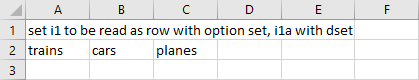Either of the following two statements below reads the second row of set elements from the spreadsheet above:

gdxxrw exampleData.xlsx  set=i1  rng=readingSets!A2:C2 cDim=1
gdxxrw exampleData.xlsx dSet=i1a rng=readingSets!A2:C2 cDim=1


When the output file is not specified, the output file will be derived from the input file by changing the file extension of the input file and removing any path information. Since all elements in the second row are unique, there is no need of increasing the maxDupeErrors parameter to avoid an error message when defining the symbol as set. By specifying the symbol directly as a dSet (domain set) in the second statement, duplicate labels would be removed without throwing an error. We set cDim to one so that the first row of the range is used for the labels of the set.

On the other hand if we want to read set elements listed in a column: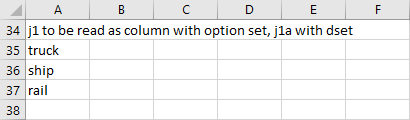Either of the following two statements reads column A of set elements from the spreadsheet above:

gdxxrw exampleData.xlsx  set=j1  rng=readingSets!A35:A37 rDim=1
gdxxrw exampleData.xlsx dSet=j1a rng=readingSets!A35:A37 rDim=1


Besides the range we also had to change the parameter rDim to indicate that the first column of the range is to be used for the labels.

This example is also part of the GAMS Data Utilities Library, see model [GDXXRWExample18] for reference.

## Reading Set and Explanatory Text

Suppose we want to read the set elements in the ninth row and their associated text in the tenth row of the following spreadsheet:Set with explanatory text stored in spreadsheet readingSets of input file exampleData.xlsx

We can read the set elements and their explanatory text by executing the command:

gdxxrw exampleData.xlsx set=i3 rng=readingSets!A9:E10 cDim=1


To read the explanatory text, we simple include the tenth row within the range of the symbol i3 and specify cDim=1. By doing this, the first row of the range, i.e. the elements of the ninth row, will be used as the set elements, while the tenth row will be interpreted as their associated text (depending on the values option specified. By default, the values option is set to dense in this example, i.e. all elements will be included and the string in the associated data cell will be used as explanatory text. See also Reading set elements associated with Data or Text for instance.).Set i3 with explanatory text displayed in GAMS Studio

Note here the explanatory text of the set element skipme2 is just a Y as it has a blank entry for the explanatory text.

This example is also part of the GAMS Data Utilities Library, see model [GDXXRWExample18] for reference.

## Reading Set Elements associated with Data or Text using the values Option

When reading set elements from spreadsheet, the values option can be used to control whether elements and associated text are included in the set or not. We use the data displayed in the spreadsheet below to demonstrate the yn, dense, sparse and noData specifications:The set element names are stored in the first row, the associated data cells in the second row.

1. values=yn
Run the following command to load those element names associated with nonzero data or yes without storing the data as explanatory text:

gdxxrw exampleData.xlsx set=A rng=readingWithValues!A1:M2 cDim=1 values=yn


The set A will contain the elements a, b, e, f, h, i, k and m, since the elements c, d, g, j and l are associated with a zero, a blank or a no resp. N (case insensitive).

2. values=dense
This option must be specified, if we want to read all elements while using the strings in the data cells as explanatory text.

gdxxrw exampleData.xlsx set=A rng=readingWithValues!A1:M2 cDim=1 values=dense


3. values=sparse
To read in all elements having a non-empty data cell while interpreting the string in the data cell as explanatory text, run the following command:

gdxxrw exampleData.xlsx set=A rng=readingWithValues!A1:M2 cDim=1 values=sparse


The set A will contain all elements except for j, since the associated data cell is empty.

4. values=noData
This option must be used, if we want to read all set elements while ignoring the data range. Especially, the data cells will not be interpreted as explanatory text. To read the elements from the range A1:M1, one could run the following command:

gdxxrw exampleData.xlsx set=A rng=readingWithValues!A1:M1 cDim=1


While all elements a - m will be included this way, the second row will be automatically interpreted as explanatory text (even though we specified only the first row within the rng statement). This might be not desirable at all in some situations, e.g. when reading the city names in the next example Reading Set from Data Tables, we do not want to have the numbers 5000, 6000 and 0 to be explanatory text for the city names. Run the following command to include all elements in your set without interpreting the cells in the second row as explanatory text:

gdxxrw exampleData.xlsx set=A rng=readingWithValues!A1:M1 cDim=1 values=noData


5. values=auto (default)
The second table within the description of the values options indicates which value type will be used by default based on the rng, cDim and rDim specifications for the set. For instance, when running the following command:

gdxxrw exampleData.xlsx set=A rng=readingWithValues!A1:M2 cDim=1


the value type used is dense, since we specified a block range with a data row and rDim equals zero.Results for yn, dense, sparse and noData; from the left to the right

This example is also part of the GAMS Data Utilities Library, see model [GDXXRWExample9] for reference.

## Reading Set from Data Tables

One may wish to load set elements from a data table. Given a spreadsheet segment like the following: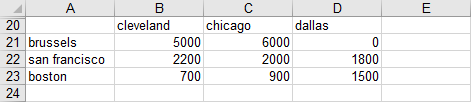We can take the set across the top containing the elements cleveland, chicago and dallas with any of the following commands:

gdxxrw exampleData.xlsx  set=i6  rng=readingSets!B20:D20 cDim=1 values=noData
gdxxrw exampleData.xlsx dSet=i6a rng=readingSets!B20:D20 cDim=1
gdxxrw exampleData.xlsx  set=i6c rng=readingSets!B20:D21 cDim=1 values=noData


Note the usage of the values option in order to avoid reading the numbers as explanatory text. See also Reading set elements associated with Data or Text. However, this can also be avoided by declaring the symbol as a domain set using the symbol declaration dSet.
We can also take a set vertically from column A as follows:

gdxxrw exampleData.xlsx dSet=j4 rng=readingSets!A21:A23 rDim=1


This example is also part of the GAMS Data Utilities Library, see model [GDXXRWExample18] for reference.

## Reading Set from Lists with Duplication

One may wish to extract set elements from a spreadsheet where there is no unique list of elements that can be read but rather a list where some names are repeated. In the example below note that in rows 26 and 27 there are set element names but they are duplicated:Spreadsheet readingSets of input file exampleData.xlsx with duplicate set elements

One can read this using dSet as follows:

gdxxrw exampleData.xlsx dSet=i7 rng=readingSets!B26:E26 cDim=1 dSet=i8 rng=readingSets!B27:E27 cDim=1


Both domain sets will be read within a single GDXXRW call. The rng and cDim specifications affect only the symbol that they are following directly.

It may be favored in some situation to use the set symbol instead (e.g. for reading explanatory text). To oppress an error message when reading sets with duplication, one must specify a sufficient large number within the maxDupeErrors option.

gdxxrw exampleData.xlsx maxDupeErrors=4 set=i7 rng=readingSets!B26:E26 cDim=1 values=noData set=i8 rng=readingSets!B27:E27 cDim=1 values=noData


For the data in this example, four is a sufficient large number since there are two duplicates for the first and two duplicates for the second set within each range. Note the usage of the values option in order to avoid reading 'cleveland' as explanatory text for the elements of set i7 and to avoid reading the numbers as explanatory text for the elements of i8. See also Reading set elements associated with Data or Text for more informations about the values option.

This example is also part of the GAMS Data Utilities Library, see model [GDXXRWExample18] for reference.

Assuming we want to read parameter data1 from the file Test1.xlsx and write the data to Test1.gdx.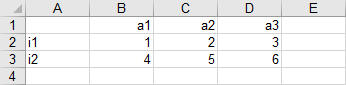First sheet of input file Test1.xlsx

The following statement reads parameter data1 from the spreadsheet above (using the par data type):

gdxxrw Test1.xlsx par=data1 rng=A1:D3 cDim=1 rDim=1


The sheet name in a range can be omitted when it refers to the first sheet. The elements in the first row and first column of the data range will be used as labels for the two dimensional parameter data1 by defining cDim=1 and rDim=1 (see also cDim resp. rDim).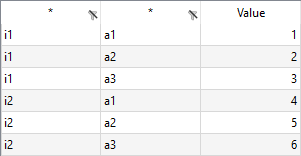Parameter data1 displayed in GAMS Studio

This example is also part of the GAMS Data Utilities Library, see model [GDXXRWExample5] for reference.

## Reading Parameter from Spreadsheet with Duplication

The same data as in the previous example, but organized differently. We use the dSet symbol specification instead of set to read set I (in column A) and set A (in column B), since there are duplicate entries in column A resp. column B.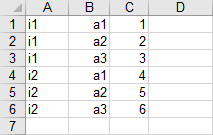Sheet EX2 of input file Test1.xlsx

The following statement reads parameter data2 from the spreadsheet above:

gdxxrw Test1.xlsx par=data2 rng=EX2!A1 rDim=2 dSet=I rng=EX2!A1 rDim=1 dSet=A rng=EX2!B1 rDim=1


By setting rDim=2 for the parameter data2 we indicate to use the first two columns of the data range as the labels for the parameter values. Since the sheet does not contain further data, one can specify the ranges using the top left cell notation without hesitation.
When using a few symbols, the command line can become too long to be practical. In such case, use a text file to hold the parameters. A parameter file can contain multiple lines to increase readability and a line starting with a '*' will be ignored.

* file example6.txt
par =data2 rng=EX2!A1 rDim=2
dSet=I     rng=EX2!A1 rDim=1
dSet=A     rng=EX2!B1 rDim=1


An option file is indicated by preceding the file name with a @ (At sign.).

gdxxrw Test1.xlsx @example6.txt


This example is also part of the GAMS Data Utilities Library, see model [GDXXRWExample6] for reference.

Note
• An option file can contain multiple lines to increase readability.
• When reading parameters from a text file, lines starting with an asterisk (*) will be ignored and act as a comment.
• An option file can also be written during the execution of a GAMS model using the GAMS Put Facility and the subsequent GDXXRW commands must use execute command so the put file is written before it is to be read (this would happen when using the compile time command $call to run GDXXRW). • Using an option file can be useful in reducing execution time by combining the options and symbols from multiple GDXXRW calls in a single option file used in a single GDXXRW call. ## Reading Multi-dimensional Parameter from Spreadsheet This example illustrates how to read a four dimensional parameter from spreadsheet: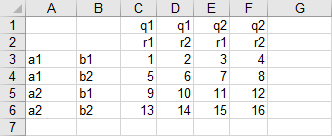Sheet EX3 of input file Test1.xlsx The strings in the first two columns and the first two rows of the data range A1:F6 shall be used as labels for the parameter values. Therefore, we define rDim=2 and cDim=2 (see also rDim and cDim). The parameter will be named data3 (within the par declaration). Run the following command to read the data with GDXXRW: gdxxrw Test1.xlsx par=data3 rng=EX3!A1:F6 rDim=2 cDim=2  Note that the data range was specified using the block range notation. However, it might be more comfortable to specify only the top left corner sometimes, but empty rows or columns may affect the reading process, i.e. GDXXRW might stop to early when encountering empty rows or columns or it will try to read data separated by empty rows or columns not being part of the data you wish to read. When we specify the range as a block, an empty row or column will always be ignored. When we specify the top left cell only, the skipEmpty option can be used to ignore one or more empty rows or columns. However, for the data in this example, we do not need to ignore empty rows or columns within the data range, but suppose there is non-relevant data starting in column H. By default (skipEmpty=1), GDXXRW would try to read the data starting in H. When we specify skipEmpty=0 and cells A7, B7, G1 and G2 are empty, the range can be specified with the top left cell only in this example: gdxxrw Test1.xlsx skipEmpty=0 par=data3 rng=EX3!A1 rDim=2 cDim=2  Since skipEmpty is a global option, affecting every symbol that follows, we define it before declaring the parameter data3. This example is also part of the GAMS Data Utilities Library, see model [GDXXRWExample7] for reference. ## Reading Spreadsheet using the Index Option The index option is used to read a number of parameters and sets based on information stored in the spreadsheet itself. By doing this, the GDXXRW command becomes quit short and the informations on how to read the data can be written directly within the spreadsheet to increase readability. The first row of the range is used for column headings indicating additional parameters. We will discuss the results only briefly, because all other options used in this example were demonstrated before. Suppose we want to read the parameters and sets from the following spreadsheet: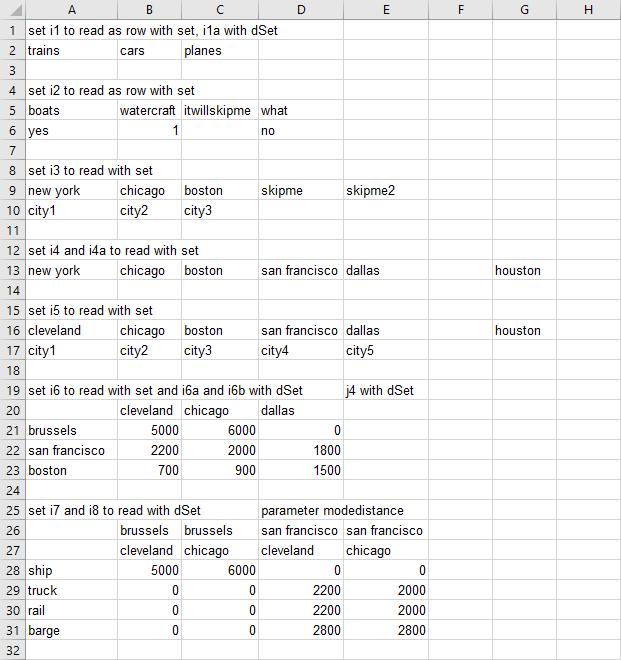Spreadsheet testIndex of input file exampleData.xlsx The informations about all parameters and sets are stored within the sheet index in the same spreadsheet file exampleData.xlsx as testIndex above: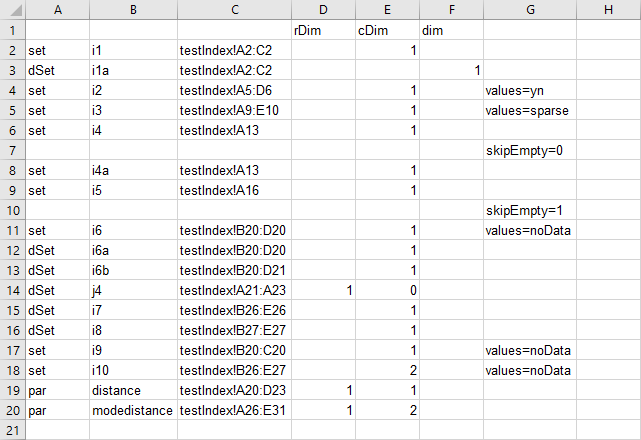Spreadsheet index of input file exampleData.xlsx The following statement reads parameters and sets from the spreadsheets using the index option: gdxxrw exampleData.xlsx output=gdxAll.gdx index=index!A1  We use the output option to write the data to gdxAll.gdx for demonstration here. By default, GDXXRW would write to testIndex.gdx. Some brief remarks on the results: • Elements of set i1 and i1a: trains, cars and planes. There is no explanatory text, since the data range (third row) is empty. We can use dim=1 equivalently to cDim=1 in this example, because by default, cDim=1 and rDim=dim-1. See also dimensions. • When reading the sets i2 and i3, we are defining which elements and explanatory text will be stored by specifying the values option. • The set i4 will contain all elements of the thirteenth row. Afterwards, skipEmpty is set to zero, affecting the reading of i4a and i5. Unlike i4, the sets i4a and i5 will not contain the element houston, since skipEmpty=0 and the empty column signals GDXXRW to stop reading (note that the range is not defined using the block range specification). Since there is a data range for i5, the strings city1 - city5 will be used as explanatory text by default. After reading i4a and i5, skipEmpty is reset to zero (default). • Each of the sets i6, i6a and i6b will contain the elements cleveland, chicago and dallas. There is no explanatory text for any of the sets in the GDX file, however, one must enforce this for the set i6 with values=noData. There are no interesting details for the remaining sets and parameters to discuss here. The complete example and the results displayed within GAMS can be found in the GAMS Data Utilities Library, see model [GDXXRWExample10] for reference. Note • The parameters and sets are read using the specifications within the myIndex sheet. They are treated as if they appeared directly on the command line. • In the spreadsheet, the first three columns of the range have a fixed interpretation: DataType (par, set, dSet, equ, or var), Item name identifier and spreadsheet data range. The fourth and following columns can be used for additional parameters like dim, rDim, cDim, merge, clear and skipEmpty. The column header contains the keyword when necessary, and the cell content is used as the option value. • When an entry appears in a column without a heading then it is directly copied into the GDXXRW option file. Thus in the example above the items in column G are directly copied into the file. • Rows do not need to have entries in the first three columns if one just wants to enter persistent options such as skipEmpty or some of the special character string re-definitions (as in row seven and ten from the spreadsheet above). ## Reading Data from Spreadsheet and Loading into GAMS One can use $call to execute the GDXXRW command in the GAMS code to read from spreadsheet at compilation time (the data is taken from the previous example):

$call gdxxrw testIndex.xlsx set=i9 rng=Sheet1!B20:C20 cDim=1 values=noData  Getting a set from the spreadsheet into a GDX file is only half the battle. One must also use commands in GAMS to load the data as discussed in the chapter Using GAMS Data Exchange or GDX Files. At compile time this is done using: Set i9; * read/load set from data at compile time$call gdxxrw testIndex.xlsx set=i9 rng=Sheet1!B20:C20 cDim=1 values=noData
$gdxIn testIndex.gdx$load i9


where the set must be declared in a set statement then one can if needed create the GDX file using GDXXRW, then one uses a $gdxIn to identify the source file and a $load to bring in the data.

Some users may wish to load sets at execution time. However, this is limited to subsets that are dynamic sets and cannot be used in domains. To do this one simply uses the statements as above, but substitutes execute in place of $call as follows: Set i9(i6a); * read/load set from data at execution time execute 'gdxxrw testIndex.xlsx set=i9 rng=Sheet1!B20:C20 cDim=1 values=noData' execute_load 'testIndex' i9;  where the set i9 must be declared as a subset in a set statement (of i6a in this case), then one can if needed create the GDX file using execution time GDXXRW, and an execute_load to bring in the data with an identification of the GDX source file name. Note that we used the set ì6a as superset, fitting best to the data from the previous example. However, one could also use the statement Set i9(*);. One can load the universe of labels from a GDX file into a set at run-time using the syntax: execute_load 'someFile', someSet=*;  Note In doing this, only labels known to the GAMS program will be loaded. ## Reading empty Cells with colMerge Suppose we want to read the four dimensional parameter from the following spreadsheet: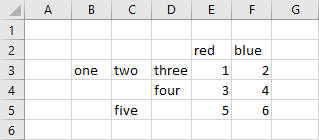Spreadsheet colMerge of input file exampleData.xlsx The cells B4, B5, C4 and D5 might be empty to avoid duplication, i.e., the non-empty content of the previous cell in the same column shall be used as content for the empty cell. In particular: the content of B3 shall be used for the content of B4 and B5, the content of C3 for C4 and the content of D4 for cell D5. Reading the above spreadsheet using the following GAMS statement: gdxxrw exampleData.xlsx par=A_d rng=colMerge!B2 rDim=3 cDim=1  results in empty cells B4, B5, C4 and D5, causing troubles if you want to declare the parameter as A(number,number,number,color) certainly: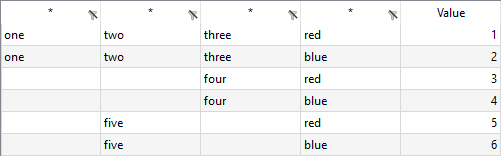GDX file with empty UELs for the first three dimensions displayed in GAMS Studio Adding the symbol attribute colMerge, we use the non-empty content of the previous cell in the same column as the content for the empty cell. Specifying colMerge=2 will do this for the first two columns for instance. gdxxrw exampleData.xlsx par=A_2 rng=colMerge!B2 rDim=3 cDim=1 colMerge=2  Only the two entries corresponding to the cell D5 are still empty, since we do not specify all three columns within colMerge:GDX file with colMerge=2 displayed in GAMS Studio Note A blank field displayed in GAMS Studio indicates an empty UEL. In the GAMS IDE, there would be an <empty> entry instead. This example is also part of the GAMS Data Utilities Library, see model [GDXXRWExample20] for reference. ## Reading merged Excel Ranges with cMerge Suppose we want to read the three dimensional parameter from the following spreadsheet:Spreadsheet cMerge with merged cells of input file exampleData.xlsx Note that the label 'red' is centered over the merged cells B1, C1 and D1 and label 'green' over the merged cells E1 and F1. Additionally, in the data range, the cells B3:C3 and B4:C4 are merged, too. The option cMerge can be used to control the way merged cells are handled. We will discuss the effect of the possible values for cMerge on the data presented above by running the following commands (an option file is used to increase readability): $onEcho > howToRead.txt
cMerge=0 par=B_d rng=cMerge!A1 rDim=1 cDim=2
cMerge=1 par=B_1 rng=cMerge!A1 rDim=1 cDim=2
cMerge=2 par=B_2 rng=cMerge!A1 rDim=1 cDim=2
$offEcho$call gdxxrw exampleData.xlsx output=cMerge.gdx @howToRead.txt


We specify cMerge in advance, since it is a global option affecting every symbol that follows. Executing the three statements will create three different output files, all displayed in GAMS Studio:From left to the right: cMerge=0, cMerge=1, cMerge=2
Note
A blank field displayed in GAMS Studio indicates an empty UEL. In the GAMS IDE, there would be an <empty> entry instead.

Some remarks on the results:

cMerge=0 (default)
Empty cells being part of a merged Excel range will remain empty. Thus, the cells C1, D1, F1, C3 and C4 from the spreadsheet above will remain empty when reading with GDXXRW. Since C3 and C4 are empty while being part of the data range, they won't show up in the GDX file. The values in the last column of the GDX file are useful to compare the results with the spreadsheet. For instance, the UELs of the value 5 are a, <empty> and two, since the cell F1 is empty, while A3 contains the string a and F2 the string two.

cMerge=1
The value of a merged range within a row or column header will be used for all cells being part of the merged range. Thus, the string 'red' will be used for the cells in the column header C1 and D1 and the string 'green' for the cell F1. Since C3 and C4 are part of the data range, they will remain empty and are not displayed in the GDX file. As you can see, there is no longer an empty UEL.

cMerge=2
The value of a merged range will be used for all cells being part of the merged range, i.e. cMerge=1 is extended to the data range. Therefore, the value 1 resp. 11 will be also used for the cell C3 resp. C4, appearing in the GDX file for the first time. Since there is no change in handling merged cells within the row or column header, all values have non-empty labels.

This example is also part of the GAMS Data Utilities Library, see model [GDXXRWExample20] for reference.

## Skipping Empty Rows and Columns

By using the skipEmpty option, we can control the way blank rows or columns are handled and causes GDXXRW to either stop or skip over if a blank row or column is encountered when using the top left corner range specification instead of a block range. If the range is specified using the TopLeft:BottomRight cell notation (often refered as block range notation), empty rows or columns will be skipped automatically. Suppose the data is stored in the following spreadsheet:Sheet skippingRC of input file exampleData.xlsx

We can read this spreadsheet and skip blank rows and columns with the following command:

gdxxrw exampleData.xlsx      par=A_d rng=skippingRC!A2 rDim=2 cDim=1


or

gdxxrw exampleData.xlsx se=1 par=A_1 rng=skippingRC!A2 rDim=2 cDim=1


Note that there will be no difference concerning the generated GDX files, since skipEmpty is set to one by default. On the other hand, if skipEmpty is set to zero

gdxxrw exampleData.xlsx se=0 par=A_0 rng=skippingRC!A2 rDim=2 cDim=1


the blanks terminate the read not reading the rail column and the san francisco.chicago row. After loading into GAMS the data become: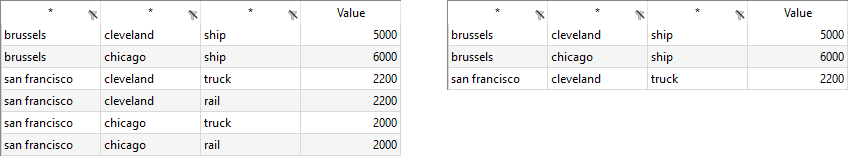Results for skipEmpty=1 on the left and skipEmpty=0 on the right

This example is also part of the GAMS Data Utilities Library, see model [GDXXRWExample21] for reference.

Note
The skipEmpty option must appear before any par, set, dSet etc statements that use it and will persist for the rest of the statements in a command unless it is set to another value.

## Ignoring Rows and Columns

We can use the options ignoreColumns and ignoreRows to ignore columns and rows when reading data for a symbol. Suppose we want to ignore the red colored rows and columns of the following spreadsheet, when reading the four dimensional parameter:Red columns and rows in the spreadsheet ignoringRC shall be ignored

We can read this spreadsheet and ignore the red colored columns and rows with GDXXRW by running the following command:

gdxxrw exampleData.xlsx par=A rng=ignoringRCC1 cDim=2 rDim=2 ignoreRows=2,6 ignoreColumns=D,G


The options ignoreRows and ignoreColumns are symbol options and therefore must appear after the symbol specification, affecting only this particular symbol.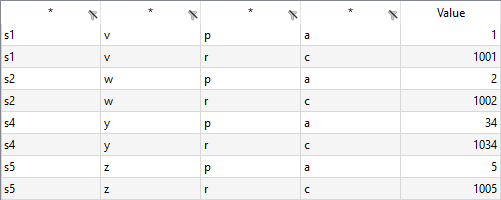Parameter A displayed in GAMS Studio

In the example above we ignored column D which would have been part of the index for the rows. So the range for the row index was extended with column E. The E column is no longer part of the data range. The treatment of the column index is similar. The second row would have been part of the column index, and now that the row is ignored, the next row becomes part of the column index and the third row is no longer part of the data range.

This example is also part of the GAMS Data Utilities Library, see model [GDXXRWExample21] for reference.

Note
The ignoreColumns and ignoreRows options appear after any par, set, dSet etc GDXXRW command instruction and only affect reading of that item.

## Reading Parameter from Spreadsheet using pre-defined Excel Named Ranges

As mentioned in section Excel Ranges, the range of a symbol to be read can be defined by using named ranges. To name a cell range within Excel, simply select the cell range and type in the name you want to assign to this particular range by using the name-box next to the top left corner of your spreadsheet. Suppose we want to read the data in the range A1:D3 taken from the example Reading Parameter from Spreadsheet:Named range 'parRange'

Instead of specifying the range explicitly by rng=A1:D3, we use the pre-defined named range 'parRange', i.e. rng=parRange. GDXXRW uses the string specified to search for a matching pre-defined named range first. In summary, run the following command to read the parameter data4 from the file Test1.xlsx:

gdxxrw Test1.xlsx par=data4 rng=parRange rDim=1 cDim=1


This example is also part of the GAMS Data Utilities Library, see model [GDXXRWExample5] for reference.

Note
In Excel, one can assign a single name to several separated block ranges, e.g. assign the name 'disconnected' to the block ranges A1:D3 and F4:H5 (by holding 'Strg' while selecting the second block range and using the name-box to assign the name). However, such disconnected data cannot be read using the named-range specification.

# Writing to Spreadsheet - Examples:

When writing to spreadsheet with GDXXRW, one must use commands in GAMS to place the data into the GDX file at first (see also Using GAMS Data Exchange or GDX files). When reading data, it is often desirable to use a $call command to run GDXXRW and the statements $gdxIn and $load afterwards to load the data, allowing also domain definitions (at compile time) for instance. This is hardly ever desirable or realizable when unloading and writing to a spreadsheet, for example, if one wish to write the solution after solving a model to spreadsheet. This should generally not be done at compile time so one should only use the execute and execute_unload commands at execution time as follows: execute_unload 'test.gdx' someParameter; execute 'gdxxrw test.gdx par=someParameter rng=A1'  where the execute_unload tells what data to place in the GDX file and determines the GDX source file name. The matching GDXXRW execution tells the name of the GDX file, the name of the spreadsheet (optional) and identifies the data to write. See also Writing Set to Spreadsheet and Writing Parameter to Spreadsheet for demonstration. Note • One must be careful when using GDXXRW as each time the command is executed the GDX file is erased and only has the current contents and thus should be written just before if reusing the name. • One also obtains output of sets using the command execute_unloaddi where the GDX file automatically includes all sets associated with unloaded parameters, variables and equations without need to list the set element names. ## Writing Set to Spreadsheet At fist, we will create a GDX file containing a simple set using the execute and execute_unloading directives. Most of the elements have an explanatory text: Set x / element1 'explanatory text' element2 element3 'previous element does not have explanatory text' /; execute_unload 'writingSet.gdx' x;  Of course, in this particular code section above, one could also use $gdxOut and $unload. The values option can be used to control whether explanatory text is written to the spreadsheet. We'll demonstrate all three possible values explicitly within a single GDXXRW execution: $onEcho > howToWrite.txt
hText="values: noData,,,values: yn,,,values: string" rng=Sheet1!A1
set=x rng=Sheet1!A2 rDim=1 values=noData
set=x rng=Sheet1!D2 rDim=1 values=yn
set=x rng=Sheet1!G2 rDim=1 values=string
$offEcho execute 'gdxxrw writingSet.gdx output=writingSet.xlsx @howToWrite.txt'  Before executing this example, check if the Excel file (writingSet.xlsx) is open. If you run GDXXRW for writing a file sharing conflict will arise. To avoid this problem, either close the Excel file or use the Excel Tools menu to make this a shared notebook. After writing to the spreadsheet (still opened), use the Excel "File Save" command to verify the changes made. By adding two additional commas within the hText statement, the cells B1, C1 and E1, F1 will be skipped when writing the text to the first row of the spreadsheet. If values=noData, neither explanatory text nor a Y are written to spreadsheet for the set elements. If values=yn, GDXXRW writes a Y for each set element to spreadsheet. To write the explanatory text, specify values=string.Set x written to the first sheet of the output file writingSet.xlsx Since cDim=0, the default option is string (see values). Imagine a two dimensional set, one could write the set in a table format, i.e. cDim=1 and rDim=1. By default, GDXXRW would write this set using the values=yn format. This example is also part of the GAMS Data Utilities Library, see model [GDXXRWExample11b] for reference. Note • A workbook cannot in general be open unless you have made special provisions with an error signaled indicating a file sharing conflict will arise when the target file is open in Excel. • To avoid the sharing conflict error the user must either close the file or indicate that the spreadsheet is a shared Excel workbook by using the Excel Tools Share Workbook dialogue. • In an open shared workbook the contents are not updated until you have done a file save in Excel. • Writing to a shared workbook can be painfully slow. • In general, it is best to close the workbook. ## Writing Parameter to Spreadsheet At first, we create a GDX file, containing some random data: * file makeData.gms Set i / i1*i4 / j / j1*j4 / k / k1*k4 /; Parameter V(i,j,k); V(i,j,k)$(uniform(0,1) < 0.30) = uniform(0,1);


When we run this GAMS model from the command prompt using the following statement, the file writingPar.gdx will be created at the end of the run.

GAMS makeData gdx=writingPar


Using the file writingPar.gdx, we can write to a spreadsheet:

Write parameter V to the first cell in the first sheet; because we only specify the top left corner of the sheet, the complete sheet can be used to store the data. We do not specify the row and column dimension, so they will be set to rDim=2 and cDim=1 by default. (See also dimensions)

By using the following command (remember to close an already existing file writingPar.xlsx in advance or make it a shared notebook as discussed in the previous example):

gdxxrw writingPar.gdx output=writingPar.xlsx par=V rng=A1


The steps above can be combined in a single GAMS model using the execute_unload and execute statements as follows:

Set
i / i1*i4 /
j / j1*j4 /
k / k1*k4 /;

Parameter V(i,j,k);
V(i,j,k)$(uniform(0,1) < 0.30) = uniform(0,1); execute_unload 'writingPar.gdx', i, j, k, V; execute 'gdxxrw writingPar.gdx par=V rng=A1';  The resultant spreadsheet looks like: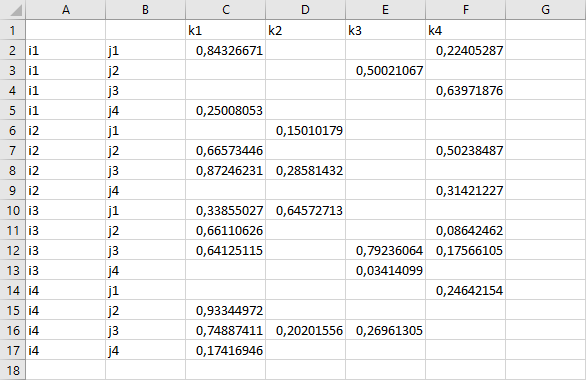Parameter V written to the first sheet of the output file writingPar.xlsx Note that if we only want to write the parameter V, there is no need to unload the sets i, j and k explicitly. The labels written to the columns A and B and to the first row are stored directly together with V in the GDX file. This example is also part of the GAMS Data Utilities Library, see model [GDXXRWExample11b] for reference. ## Writing to Spreadsheet with merge Option When writing to a spreadsheet one can control data handling and matching using the merge command line option. When merge is active, the only data that will be written to the spreadsheet are those data for which the element names match row and column labels that are in the spreadsheet already. Also under merge, spreadsheet cells for which there is no matching row/column pair will not be changed. This option might be very useful, e.g., if there is a fixed report layout/framework already in your spreadsheet file which should not be changed when writing the data from GAMS. Suppose we want to write the parameter A, already stored in data.gdx, to an existing spreadsheet:Parameter A displayed in GAMS Studio In the following spreadsheet, there are row and column labels matching most of the data in the GDX file, except for the additional column header 'horse' and the non existing row labels 'san francisco.chicago':Data already stored in the spreadsheet merge_clear of exampleData.xlsx Use the following commands to write the data from GDX to spreadsheet twice to different ranges for comparison: gdxxrw data.gdx output=exampleData.xlsx par=A rng=merge_clear!B1:G4 rDim=2 cDim=1 merge gdxxrw data.gdx output=exampleData.xlsx par=A rng=merge_clear!B8 rDim=2 cDim=1  Note that merge is a symbol option affecting only the symbol A. The resultant spreadsheet looks like:Parameter A written twice to merge_clear of exampleData.xlsx The parameter is written to the range B8-F12 without merge enabled, while the option is enabled when writing to the range B1:G4, respecting the data arrangement already existing. Note that the column and row orders vary and the san francisco - chicago row is missing since it is not mentioned in the labels within the spreadsheet before the merge operation, while the horse column is still present with it's data left alone, not being overwritten by the parameter. This example is also part of the GAMS Data Utilities Library, see model [GDXXRWExample12] for reference. Note • Using the merge option will force the data to be presented in the order in which the row and column labels are entered already. • GDX file contents that do not have matching row/column pair of named elements in the spreadsheet will be overlooked. • A write under a merge option addressing a blank area of a spreadsheet will always be blank as there will not be matching set elements at all. • The matching of labels is not case-sensitive. • Warning: Enabling the merge option will clear the Excel formulas in the rectangle used, even if the cells do not have matching row/column headings in the GDX file. Cells containing strings or numbers are not affected. ## Writing to Spreadsheet with clear Option When writing to a spreadsheet one can also use the clear option to control data handling and matching. When clear is enabled, the only data that will be written to the spreadsheet are those data for which the element names match row and column labels that are in the spreadsheet already but all data and formulas in the target range will be removed. Suppose the parameter A from the previous example is stored in data.gdx and there are row and column labels matching most of the data in the GDX file, except for the additional column header 'horse' and the non existing row labels 'san francisco.chicago':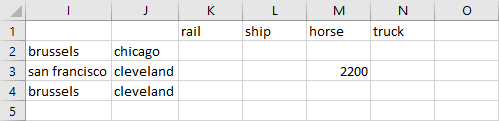Data already stored in the spreadsheet merge_clear of exampleData.xlsx Use the following command to write to exampleData.xlsx with clear enabled: gdxxrw data.gdx output=exampleData.xlsx par=A rng=merge_clear!I1 rDim=2 cDim=1 clear  then the result isParameter A written to spreadsheet with clear enabled The results are similar to those under merge but the old data in the column labeled 'horse' has been removed. This example is also part of the GAMS Data Utilities Library, see model [GDXXRWExample12] for reference. Note • Using the clear option will force the data to be presented in the order in which the row and column labels are entered already. • GDX file contents that do not have matching row/column pair of named elements in the spreadsheet will be overlooked. • A write under a clear option addressing a blank area of a spreadsheet will always be blank as there will not be matching set elements. • The matching of labels is not case-sensitive. • Warning: The clear option will clear all Excel formulas and values in the rectangle used, even if the cells do not have matching row/column labels in the GDX file. ## Writing to Spreadsheet using a Filter In Excel, you can filter the data by some specified conditions so that only the data matching the conditions is displayed. This might be useful in some cases, e.g. it helps you to focus on the most relevant data within a large table of data. With GDXXRW you can add some basic filter to your spreadsheet when writing data from a GDX file. The following example creates a small GDX file with some random data, which is used to write the symbol A to a spreadsheet later on with the filter option enabled. Set i / i1*i2 / j / j1*j2 / k / k1*k2 /; Parameter A(i,j,k); A(i,j,k) = uniform(0,1); execute_unload 'test.gdx', A; execute 'gdxxrw test.gdx filter=1 par=A rDim=1 cDim=2 rng=Sheet1!A1';  Since filter is a global option, it must be specified in advance of the symbols for which you want to add a filter. The default value is zero, i.e. no filter will be added. If there are multiple rows in the column header, i.e. cDim is greater than zero, the valid range for the filter option is 1..cDim.Parameter A written to spreadsheet with filter=1 The screenshot above shows the filter in Excel. When we specify filter=2 in this example with two dimensions for the column header, the row with the filter moves away from the data range as illustrated below:Parameter A written to spreadsheet with filter=2 One could now filter the data, e.g. displaying only the values where the label of the first dimension is i1 by selecting this value exclusively within the drop down menu of column A. This example is also part of the GAMS Data Utilities Library, see model [GDXXRWExample15] for reference. ## Writing to Spreadsheet adding Text and Hyperlinks The following example illustrates the use of the text directive. Adding text and hyperlinks to your spreadsheet is useful to customize the output and to navigate more quickly through the data. First, we write some data to a GDX file and we use the text directive to write text to various cells in the spreadsheet; some of the cells are hyperlinks to other locations. To increase readability, we'll use a parameter file howToWrite.txt to shorten the GDXXRW statement. Set i / i1*i9 / j / j1*j9 /; Parameter A(i,j); A(i,j) = 10*ord(i) + ord(j); execute_unload 'pv.gdx' A;$onEcho > howToWrite.txt
text="Below the data for symbol A" rng=data!C2
par=A rng=data!C4
text="Back to index" rng=data!A1 link=Index!A1
$offEcho execute 'gdxxrw pv.gdx output=pv.xlsx @howToWrite.txt'  We will write the text "Link to data" to the cell A2 of sheet Index. The option linkID is used to add a hyperlink to the range of the symbol A. In addition, we create a hyperlink "Back to index" in the cell A1 of sheet data using the link option pointing to the cell A1 of sheet Index. One can also specify links to external sources. For demonstration, we add a link to the GAMS homepage. Below a screen shot showing both sheets data and Index created by the commands above:Output file pv.xlsx: Adding text and hyperlinks to spreadsheet when writing This example is also part of the GAMS Data Utilities Library, see model [GDXXRWExample16] for reference. # Reading and Writing, Extended Use - Examples: ## Reads a Table from Spreadsheet, manipulates the Data and writes back to Spreadsheet In the following example, we read data from a spreadsheet and save the data in a GDX file. Using the $gdxIn and $load GAMS directives, we load the data from the GDX file into GAMS afterwards. The GAMS program modifies the data and at the end of the run the data is saved in a new GDX file (tmp.gdx). The last step updates the spreadsheet with the modified parameter.Spreadsheet test1.xlsx We read the spreadsheet and load the data from the resultant GDX file at compile time. The data modification and the unloading and writing process are done at execution time, using the execute_unload and execute directives: $call gdxxrw test1.xlsx dSet=I rng=A2:A3 rDim=1 dSet=A rng=B1:D1 cDim=1 par=X rng=A1:D3 rDim=1 cDim=1
$gdxIn test1.gdx Set I(*), A(*);$load I A

Parameter X(I,A);
$load X display I, A, X;$gdxIn
X(I,A) = - X(I,A);

execute_unload 'tmp.gdx', I, A, X;
execute 'gdxxrw tmp.gdx output=test1.xlsx par=X rng=EX6!A1:D3 rDim=1 cDim=1';


The parameter is written to the sheet EX6. However, since we only write the parameter X, we do not necessarily have to unload the set I and A.

This example is also part of the GAMS Data Utilities Library, see model [GDXXRWExample13] for reference.

## Reading Data from Spreadsheet and writing Data to Spreadsheet after Solve

In this example, we use a modified version of the [trnsport] model from the GAMS model library to demonstrate the process of reading data, defining and solving the model and writing a solution report to spreadsheet altogether. This example illustrates in particular:

• Compilation phase
• Read data from a spreadsheet and create a GDX file
• Loading sets from the GDX file
• Using the sets as a domain for additional declarations
• Execution phase
• Solve the model
• Write solution to a GDX file
• Use GDX file to update spreadsheetData for trnsport model

A parameter file howToRead.txt is used to increase the readability of the GDXXRW call. Note that the dimension of the scalar we want to read is set to zero. The range of each parameter can be specified by using the top left corner only, since there are two empty rows separating the data blocks from each other and the default value of skipEmpty signals to stop reading if two empty rows occur.

$onEcho > howToRead.txt dSet=i rng=A3:A4 rDim=1 dSet=j rng=B2:D2 cDim=1 par =d rng=A2 rDim=1 cDim=1 par =a rng=A8 rDim=1 par =b rng=A13 rDim=1 par =f rng=A19 dim=0$offEcho
$call gdxxrw TrnsportData.xlsx @howToRead.txt$gdxIn TrnsportData.gdx

Set
i(*) 'canning plants'
j(*) 'markets';

$load i j display i, j; Parameter a(i) 'capacity of plant i in cases' b(j) 'demand at market j in cases' d(i,j) 'distance in thousands of miles'; Scalar f 'freight in dollars per case per thousand miles';$load d a b f
$gdxIn Parameter c(i,j) 'transport cost in thousands of dollars per case'; c(i,j) = f*d(i,j)/1000; Variable x(i,j) 'shipment quantities in cases' z 'total transportation costs in thousands of dollars'; Positive Variable x; Equation cost 'define objective function' supply(i) 'observe supply limit at plant i' demand(j) 'satisfy demand at market j'; cost.. z =e= sum((i,j), c(i,j)*x(i,j)); supply(i).. sum(j, x(i,j)) =l= a(i); demand(j).. sum(i, x(i,j)) =g= b(j); Model transport / all /; solve transport using lp minimizing z; display x.l, x.m; execute_unload 'TrnsportData.gdx', x; execute 'gdxxrw TrnsportData.gdx output=TrnsportData.xlsx squeeze=n var=x.l rng=Sheet2!A1';  The solution is written to Sheet2 of the input file TrnsportData.xlsx by executing GDXXRW at execution time. The var statement is used in the symbol specification to write out the level of variable x. In order to write zero values, the squeeze option is disabled. Otherwise, the cells C4 and D3 remain blank.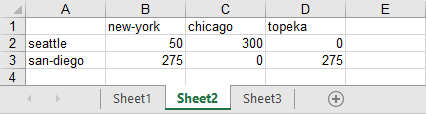Solution written to the top left corner of Sheet2 This example is also part of the GAMS Data Utilities Library, see model [GDXXRWExample14] for reference. ## Reading Special Values from Spreadsheet and writing to Spreadsheet This example demonstrates the reading and writing of special values with GDXXRW. Reading Special Values Assuming we want to read the special values stored in the spreadsheet below: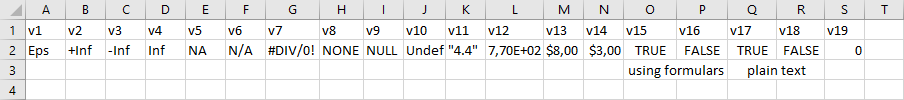Spreadsheet specialValues The following statement reads parameter vIN from the spreadsheet above: gdxxrw exampleData.xlsx output=specialValues.gdx NaIn=N/A squeeze=n par=vIN cDim=1 rng=specialValues!A1:S2  To affect the parameter vIN, the global options NaIn and squeeze (to display zero values in the GDX file) must be specified in advance.Parameter vIN displayed in GAMS Studio The cells containing the strings Eps, +Inf, -Inf, Inf, NA and Undf are read in correctly. The division by zero error in the spreadsheet will be written as Undf. We defined a new string - 'N/A' - within the option NaIn to be recognized as NA additionally. Within GAMS, there is no directly comparable data type for 'None' and 'Null', so these strings will be interpreted as Undf. Note the importance of the cell format specified within Excel. For instance, the values for v13 and v14 are different, although both fields contain a Dollar sign, since the cell format of M2 is 'General', while the cell format of N2 is 'Currency'. The boolean 'False' turns into zero after reading, while 'True' turns into -1. Note here, that this is independent whether the booleans are written as plain text within Excel or by using the formulas '=True()' resp. '=False()'. We disabled squeeze in order to display the zero values for the elements v16, v18 and v19 in the GDX file. Writing Special Values Initially, we declare a parameter with special values and generate a GDX file from it: $onUndf
Set v / v1*v7 /;
Parameter vOUT(v) / v1 Eps, v2 +Inf, v3 -Inf, v4 Inf, v5 Undf, v6 0.0, v7 NA /;
Scalar S / 0 /;

execute_unload 'specialValues.gdx', v, vOUT, S;


We will write the parameter vOUT to spreadsheet twice. At first to demonstrate the usage of EpsOut, pInfOut, mInfOut, UndfOut, zeroOut and NaOut, the second time to show the default settings when writing. Those output string options may be useful, if the GAMS default settings are not appropriate for your Excel calculations later on or to customize the representation of the values in Excel in general. Note that there is also a scalar declaration in order to demonstrate the different behavior when writing scalars and parameter with zero values to Excel while using the zeroOut option.

$onEcho > howToWrite.txt * defining new strings to be used when writing special values: EpsOut=0 pInfOut=+1E+100 mInfOut=-1E+100 UndfOut=undefined zeroOut=zero NaOut=notAvailible * now write parameter vOUT with merge to force the column F containing set element "v6" and vOUT("v6") set=v rng=specialValues!A6:G6 cDim=1 par=vOUT rng=specialValues!A6:G7 cDim=1 merge text="Special values of Parameter vOUT written with user defined output strings:" rng=specialValues!A5 text="Scalar S / 0 /:" rng=specialValues!I6 par=S rng=specialValues!I7 * reset the strings for special values back to default und write vOUT again resetOut set=v rng=specialValues!A10:G10 cDim=1 par=vOUT rng=specialValues!A10:G11 cDim=1 merge text="Special values of Parameter vOUT written with default output strings:" rng=specialValues!A9 text="Scalar S / 0 /:" rng=specialValues!I10 par=S rng=specialValues!I11$offEcho

execute 'gdxxrw specialValues.gdx output=exampleData.xlsx @howToWrite.txt';


In order to increase readability when executing GDXXRW, we use a parameter file named howToWrite.txt and additionally, we write some text out to structure the Excel file. The range for the non-default values is A6:G7. We define the new strings to be used for the special values first, affecting the following symbols. To write the default values to the range A10:G11, we use the resetOut option to reset the output strings to default, otherwise, the new strings remain in effect, since they are global options.Excel spreadsheet specialValues after writing special values

As mentioned briefly above, the zeroOut option affects the scalar S and the parameter vOUT differently. While we get the expected result - 'zero' - for the scalar S, the cell F7 for the zero value of vOUT remains empty, since zero values of parameters are not even part of the GDX file in general (and cannot be added from your GAMS model; Note here, that the squeeze options only affects the writing of sub-fields of variables and equations). Therefore, cell F11 has no value, too. Though scalars with zero values are stored in the GDX file. We'll present a workaround for writing zero values of a parameter to spreadsheet in the next example Writing Parameter to Spreadsheet including Zero Values.

The complete example is also part of the GAMS Data Utilities Library, see model [GDXXRWExample8] for reference.

Note
• When writing to a spreadsheet, special values such as Eps, NA, Undf and Inf will be written but this can be changed. When reading data from a spreadsheet, the ASCII strings for these special character strings will be used to write corresponding special values to the GDX file.
• Cells that are empty or zero will not be written to the GDX file.

## Writing Parameter to Spreadsheet including Zero Values

There is no straight way to write zero values of a GAMS parameter to spreadsheet from your model using GDXXRW, since zero values of a parameter are not stored in the GDX file (see also the previous example Reading Special Values from Spreadsheet and writing to Spreadsheet). However, instead of the zero values one can store an EPS in the GDX file and instruct GDXXRW to use a zero when writing the value for EPS using the epsOut option afterwards as demonstrated below:

Set i / i1*i9 /;
Parameter A(i), Amod(i);
A(i) = uniformInt(0,1);

* Amod(i) = A(i) if A(i) <> 0 and Amod(i) = EPS if A(i) = 0
Amod(i) = EPS$(not A(i)) + A(i); * Unload the unmodified and modified parameter and write to spreadsheet using an option file execute_unload 'zeroPar1.gdx' A Amod;$onEcho > howToWrite1.txt
text="Parameter A" rng=A1
par=A rng=A2
text="Modified Parameter Amod written with epsOut: 0" rng=A5
epsOut=0 par=Amod rng=A6
$offEcho execute 'gdxxrw zeroPar1.gdx output=writingZeros.xlsx @howToWrite1.txt';  This approach is impracticable in the unlikely event that there are already EPS values in your parameter and you want to write these as EPS to your spreadsheet. An alternate approach regarding variables while exploiting the squeeze option is demonstrated below (parameter A and set i refer to the data above): Variable dummyPar(i); dummyPar.l(i) = A(i); * In order to write every entry of dummyPar in the spreadsheet, one must allocate * a non-zero value to one of the variable attributes .m, .lo or .up dummyPar.up(i) = 1; * Unload the dummy variable and write the .l subfield to spreadsheet while disabling squeeze execute_unload 'zeroPar2.gdx' dummyPar;$onEcho > howToWrite2.txt
text="Variable dummyPar written with squeeze: n" rng=A9
squeeze=n var=dummyPar.l rng=A10
$offEcho execute 'gdxxrw zeroPar2.gdx output=writingZeros.xlsx @howToWrite2.txt';  Creating the additional variable and the allocation of a non-zero value to one of the other variable attributes are the drawbacks of this approach. Note that we only declared the parameter Amod to keep the original data of A untouched in order to run the code in a single model and to write A to spreadsheet, too.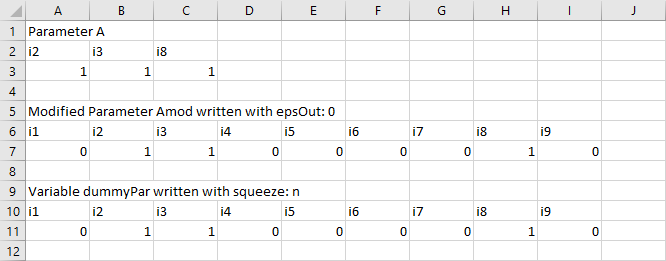Output file writingZeros.xlsx This example is also part of the GAMS Data Utilities Library, see model [GDXXRWExample19] for reference. ## Reading several Scalars from Spreadsheet Suppose you want to read a large number of scalars (i.e. scalar names and their associated values) for your model from a spreadsheet file as shown below. Originally, the data was taken from the model [indus89] of the GAMS Model Library.Spreadsheet indus89Scalars Natively, one could declare all scalars directly inside the model and read every single one of them with GDXXRW afterwards (the following code is shortened for representation): Scalar baseyear, repco;$call gdxxrw exampleData.xlsx output=indus89Scalars.gdx par=baseyear rng=indus89Scalars!B2 dim=0 par repco rng=indus89Scalars!B3 dim=0 trace=0
$gdxIn indus89Scalars.gdx$load baseyear repco


However, the code would become quit long and circumstantially for this example, especially the GDXXRW statement, because every scalar must be specified individually. Therefore, we present a more sneaky way to tackle the problem. At first, the file mkScalar.gms is written. We declare a set and a parameter to hold the scalar names and their values. A simple GDXXRW call is used to read the names and values from the file exampleData.xlsx. Afterwards, we load the data using $gdxIn and $load. The result of this is a single parameter containing the scalar values defined over a set containing the scalar names. However, this is not exactly what we are looking for. To transform this representation into several single scalar definitions, we use a simple loop over the set scalarNames and the Put Writing Facility to generate a file scalars.gms, containing those single scalar statements.

$onEchoV > mkScalar.gms Set scalarNames; Parameter scalarValues(scalarNames);$call gdxxrw exampleData.xlsx output=indus89Scalars.gdx set=scalarNames rng=indus89Scalars!A2:A22 rDim=1 par=scalarValues rng=indus89Scalars!A2:B22 rDim=1
$ifE errorLevel<>0$abort Problems reading sheet indus89Scalars with GDXXRW
$gdxIn indus89Scalars.gdx$load scalarNames scalarValues

File fs / 'scalars.gms' /;
put  fs;
loop(scalarNames, put / 'Scalar ' scalarNames.tl ' / ' scalarValues(scalarNames):>20:10 ' /;';);
$offEcho$call gams mkScalar
$include scalars.gms  Calling the file mkScalar.gms will create the file scalars.gms, containing all those scalar statements (shortened for representation): Scalar baseyear / 1988.0000000000 /; Scalar repco / 2.5000000000 /;  Finally, the file scalars.gms is included to your model. This example is also part of the GAMS Data Utilities Library, see model [GDXXRWExample17] for reference. # Changes in the Set Values Parameter The following documents some changes that were made when reading a set using the values=string option. Reading a domain or a parameter was not affected by these changes. To illustrate the various behaviors in different versions of GDXXRW, we are using the spreadsheet data as shown below, using the following call: $call gdxxrw test.xlsx set=one rng=B2 rDim=1 values=string set=two rng=B1 rDim=1 cDim=1 values=stringSpreadsheet data to illustrate Set Values

We read the one dimensional set in column B by specifying the top-left corner of the data (cell B2) or the full range (B2..B5). A two dimensional set is read using the top-left corner of the data (cell B1) or the full range (B1..E5). Variations are introduced by specifying options for values to be string or yn or noData. The option value all is only available in later versions of GDXXRW and was used to introduce the same behavior as strings in earlier versions.

## Chronological description of the changes made to the Values option:

• GAMS versions prior to version 24.3:

values=string results in reading the data dense. The contents of a cell is used for the set associated text and an element is included even if the data cell is empty.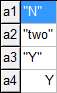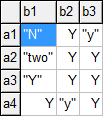• GAMS version 24.3.1

We changed the interpretation of string to mean that the set element was only to be included when the string data was not empty. Note below that element a4 is missing from the one-dimensional set and so are a1.b1, a2.b2 etc from the two-dimensional set. For the two-dimensional case this looked more or less how the GAMS compiler interprets a table statement. Unfortunately, the interpretation of empty data cells was also applied to one-dimensional sets leading to undesired results.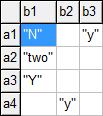• GAMS version 24.4.1

Recognizing that reading a set dense was no longer available, we introduced a new option values=all. This allowed us to read Excel data the same way as was possible before version 24.3.1 using the values=string i.e. reading the data dense and including all cells whether the data cell is empty or not and use the content of the data cell for the set associated texts.

• GAMS version 24.4.6 (Current status)

We decided to remove some confusion with the interpretation of the values option by introducing the options dense and sparse and flagging the options strings and all as deprecated. Both strings and all are replaced with dense.

Backward compatibility issues.

With these changes we broke our in house rule not to introduce changes that break backward compatibility. Because of this, the user needs to change the parameters for the GDXXRW call or change the workbook data. The parameters for the call should be changed from values=string to values=dense for one-dimensional sets where we specify the top-left corner only. In case the data in the workbook needs to be changed, inserting a string to in the data cell will address the issue. In the example on the top of the page, inserting a 'Y' in cell C5.

GAMS Development Corp.
GAMS Software GmbH

General Information and Sales
U.S. (+1) 202 342-0180
Europe: (+49) 221 949-9170